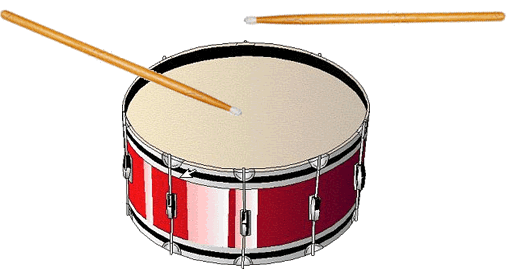Chapter 4 Subtraction is Super
Chapter 4 Subtraction is SuperWhat we learned for adding in the last chapter we now apply to subtraction. Adding is always the easier of the two, so subtraction might need a little more attention. As with the adding chapter, here we are going to focus on strategies that will help the children to be more at ease with subtraction and that let the children perform those pesky subtraction sentences in their heads. Again, we are going to demonstrate and model the strategies that the mathematicians of the world do in their heads in order to teach those who need a strategy. The same caution applies as with the adding for those that have been using some strategies already. Please just try the strategies and then use what works for you. Subtract away!!!

1.        Counting Back-

Just as with adding, counting back is a useful strategy that is to be used when the number being subtracted is 1, 2 , or 3. In class, we say “ Put the first number in your head and count back” as we actually move our hand in a backwards motion to give the visual and bodily learners a concrete picture of what is happening.

Example: 6-2=

****CAUTION****The big difference between adding and subtracting is that subtraction HAS to be done in order that it appears. Please reinforce that subtraction makes sense when the biggest number is in the front and the smallest number is at the end.

2. Do Some Math MagicOk…the minute has passed and now we are going to talk about how subtraction and addition is related. Just as with reading if you can spell “cat” you can spell “fat”, if you know the adding fact, then you know the subtraction fact. In class we say that we are doing “magic”. In fact we get out a magic wand and say, “Hocus, pocus. Alacazam…Poof!”

If you know 8 + 2 = 10 then...

you know 10 - 2 = 8REMEMBER that when doing subtraction the biggest number is ALWAYS in the FRONT.

3. We love you ten!!!

That's right ten can help us with subtraction as well. We can use a ten frame to help us subtract higher numbers like this... (drumroll please )So 15-8=  could look like this...

First put 8 dogs in the ten frame because we know that number and then add the bones to get to 15. If you count how many bones then you can see how much 15-8 is. The possibilites are just endless!Activities for Chapter 4 concepts

1.        Using Strategies to Solve Facts

Materials: 10 counters and 10 different counters (e.g. red and blue)

Procedure:

1.        Create a line of 2 red counters and 4 blue counters. Have the child separate the red counters from the blue counters and then remove the red counters from sight. Ask “How many cubes are left?”

2.      Draw a picture of what the child did by crossing out the counters.

3.      Repeat by creating a new line of counters with some red and some blue. Discuss and draw a picture for what did.

4.      Model how to count back when removing 1, 2, or 3 .

2.      Related Fact Stories

Materials: 12 counters, cup

Procedure:

1.        One person writes a number from 1 to 12 on paper and counts out that many counters. That person then hides some of the counters under the cup.

2.      The partner uses the number on the paper and the number of counters that are still out to determine the number of counters hidden under the cup. The partner describes how he found the missing number.

3.      Check the counters under the cup.

4.      Reverse roles.

As the child is figuring out how many are under the cup try and have her use the adding sentence or how many they started with to help figure out how many are in the cup. For example, if there were 10 counters to start and some are covered and there were 4 left, ask “4 plus how many will get you 10?”